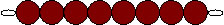# Bead Bars Multiplication

## Contents

6-9.

### Materials

• Box containing colored bead bars 1-10, 55 of each
• Chart I (for control)

### Presentation

1. We are going to represent the table of a certain number with bead bars.

2. The child is invited to choose a number, e.g., 8.

3. We start with 8 taken one time. One 8 bar is laid horizontally 8 x 1 = ?

4. The product is also represented by one 8 bar (laid vertically below the first) The child writes 8 x 1 = 8.

5. Now take 8 two times. The two 8 bars are laid horizontally 8 x 2 = ?

6. A ten bar and a six bar to represent the product are laid vertically, thus making a double row.

7. The child writes the equation in his notebook. 8 x 2 = 16

8. This continues until 8 x 10 = 80.

9. Observe the geometric figures which have been formed with the 8 bars:

8x1 is a line:and so on...

### Points Of Interest

Notice the rectangles that come before the square have a base longer than the height.

The rectangles that come after the square have a base which is shorter than the height.

### Purpose

Direct Aim:

• To help the child with the memorization of the multiplication tables.
• To bring the child to awareness of the functions of the multiplier and the multiplicand.

Indirect Aim:

•  To understand that a number when multiplied by ten results in the same number of tens and zero units.
• To realize that a number multiplied by itself results in a square to give the concept of forming surfaces, starting with a line, progressing to rectangles